# Using the Tau-R Correction on DSC Data

In Differential Scanning Calorimetry, the temperature change of a sample in a crucible is measured during heating and compared to a reference (empty crucible). The heat flow is calculated on the basis of this temperature difference curve. If the sample and reference are the same, then equal heat flows are passing through both the reference and the sample, which means that the heat flow difference is zero. When the sample changes state (e.g. melting, evaporation, crystallization, etc.) due to heating (or also cooling), this is detected as a difference in temperature and the DSC curve shows a difference in heat flow. An example for such a DSC signal is the melting of a sample depicted in Figure 1. An endothermal effect can be measured for melting.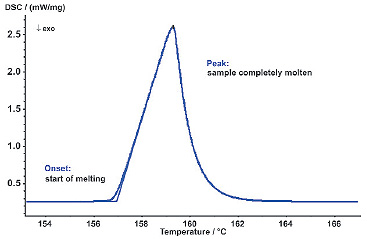Figure 1. Endothermal effect for the melting process

## Interpreting DSC Data

On closer examination of the measured DSC data, it becomes clear that the curve shape does not exactly reflect the heat flow occurring during a phase transformation of the sample. The onset temperature marks the beginning of the melting of the sample; when the peak temperature is reached, the sample is completely molten.

The peak temperature does not correspond to the melting temperature of the sample, although the sample temperature should be the same as the melting temperature. Another discrepancy between the curve shape and reality is that after reaching the peak position, the signal does not immediately drop to zero. This, however, should be the case since the sample is already completely molten at this point and no longer needs any further energy. Therefore, the DSC curve evidently incorporates information not only about the sample but also from the instrument.

## Overcomimg Discrepancies in DCS Data - The Tau-R Correction

To overcome this discrepancy, the tau-R correction has been developed. This correction method is based on two essential correction factors:

• the thermal resistance R and
• the time constant tau. 2

## The Influence of Thermal Resistance on DSC Signal

The following figure illustrates the influence of the thermal resistance R on the DSC signal.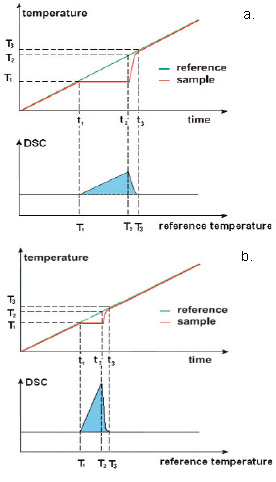Figure 2. a) poor thermal contact b) good thermal contact

If the thermal contact is poor (Fig. 2a), melting takes a longer time. As a result, the DSC curve shows a broader, lower peak when recorded against the reference temperature. If the thermal contact is good (Fig. 2b), on the other hand, Differential Scanning Calorimetry yields a sharp peak. This means that the increase in the measured signal is a function of the thermal resistance. The area below the curves, however, is equal in both cases and corresponds to the melting enthalpy.

For DSC correction, the fact that the sample temperature remains constant during the melting process is taken into consideration. The corrected DSC signal recorded against the sample temperature now shows a vertical increase in the DSC signal for melting, for example, which reflects the expected course of the melting process.

## The Influence of the Time Constant

The second parameter for the tau-R correction is the correction according to the time constant, tau. This is also an instrument-specific correction parameter. The theoretical background can be described as follows.

The heat flow signal measured by means of DSC does not show only the heat released (or used) from the sample. In addition, the heat flow is also dependent upon the heat transfer as an instrument function.

The measured signal F(t) can be depicted as the following mathematical function: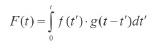where f(t) delivers the percentage of heat released from the sample.

g(t) is the heat flow as a function of the instrument and can be described with the help of an exponential function of the form g(t) = exp (-t/tau).

## The Influence of the Tau Time Constant on the DSC Signal

To illustrate the influence of the tau time constant on the DSC signal of a sample, Figure 3 shows measured DSC signals with tau time constants of differing size.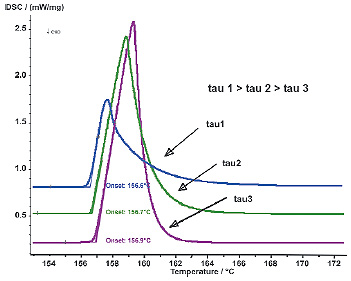Figure 3. Influence of the time constants tau on the DSC signals.

For a corrected measuring curve, the signal to the right of the peak position should equal zero, i.e. no more heat is being released or used. The result of such a correction is that the influence of the measuring instrument on the peak shape is eliminated and information is therefore only gathered about the sample and the processes occurring during phase transition.

The red curve in Figure 4 represents an uncorrected DSC measuring curve for a melting process. The curve shape shows a slow increase in the heat flow signal starting when melting begins. The maximum is reached when the sample is entirely molten. Then, the DSC signal slowly decreases exponentially toward zero, as a function of the time constants of the instrument.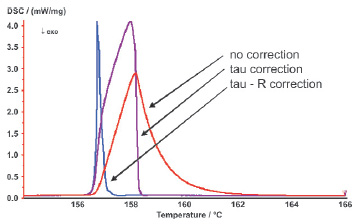Figure 4. Application of the tau-R correction.

## Corrected DSC Data

If this DSC signal is now corrected according to the tau time constant, a signal is obtained which drops back to zero immediately after reaching the peak (black curve). If the correction according to the thermal resistance, R, is now also taken into consideration (blue curve), a sharp peak is obtained for the melting process exhibiting a steep increase on its left slope and a steep decrease on its right slope. The influences on the shape of the melting peak caused by the instrument are therefore eliminated.

## Determining Correction Factors

For determination of the correction parameters tau (time constant) and R (thermal resistance), it is necessary to measure the melting process of pure metals. Based on the measuring curve obtained, the parameters can be calculated in the analysis with the NETZSCH Proteusfi software under "Evaluation" -> "Time Const. And Therm. Resistance". These parameters are determined for several metals and then saved. The calibration sets used in the temperature and sensitivity calibration can also be employed for the tau-R calibration. Under menu item "Extras" -> "tau-R Calibration", a table will be opened where these parameters are recorded and saved as a correction file. This correction file can be used to correct measurements carried out under the same conditions, such as instrument type, furnace type, sensor type, crucible type, atmosphere and purge gas.

The determination and creation of a correction file must be done only once for each instrument used, as long as the above-mentioned parameters are kept constant.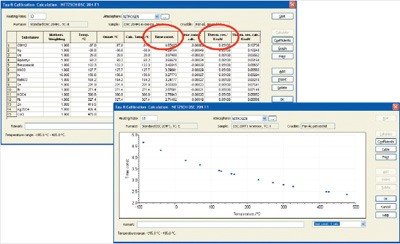Figure 5. Establishment of a tau-R correction file

## Pharmaceutical Industry

Ibuprofen is a drug classified as a non-steroidal antirheumatic agent which is employed for treating pain, inflammation and fever. DSC is an indispensable measuring method for the characterization of pharmaceuticals; the tau-R correction yields the highest in precision and correct characteristic values in this field.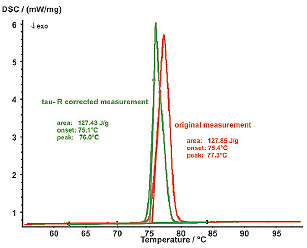Figure 6. DSC curve of Ibuprofen.

## Chemical Industry

Differential Scanning Calorimetry is used in all areas where the accurate and exact characterization of substances and materials is needed. The DSC curve in Figure 6 shows that even small and overlapping effects like the melting of Hexatriacontane can be resolved. The tau-R correction additionally allows even more precise conclusions to be drawn and yields accurate values for material characterization.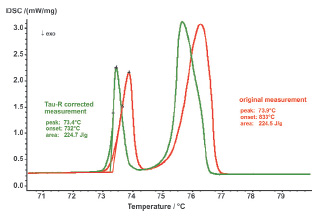Figure 7. DSC curve of Hexatriacontane.This information has been sourced, reviewed and adapted from materials provided by NETZSCH-Gerätebau GmbH.

## Citations

• APA

NETZSCH-Gerätebau GmbH. (2019, December 04). Using the Tau-R Correction on DSC Data. AZoM. Retrieved on August 10, 2020 from https://www.azom.com/article.aspx?ArticleID=4983.

• MLA

NETZSCH-Gerätebau GmbH. "Using the Tau-R Correction on DSC Data". AZoM. 10 August 2020. <https://www.azom.com/article.aspx?ArticleID=4983>.

• Chicago

NETZSCH-Gerätebau GmbH. "Using the Tau-R Correction on DSC Data". AZoM. https://www.azom.com/article.aspx?ArticleID=4983. (accessed August 10, 2020).

• Harvard

NETZSCH-Gerätebau GmbH. 2019. Using the Tau-R Correction on DSC Data. AZoM, viewed 10 August 2020, https://www.azom.com/article.aspx?ArticleID=4983.